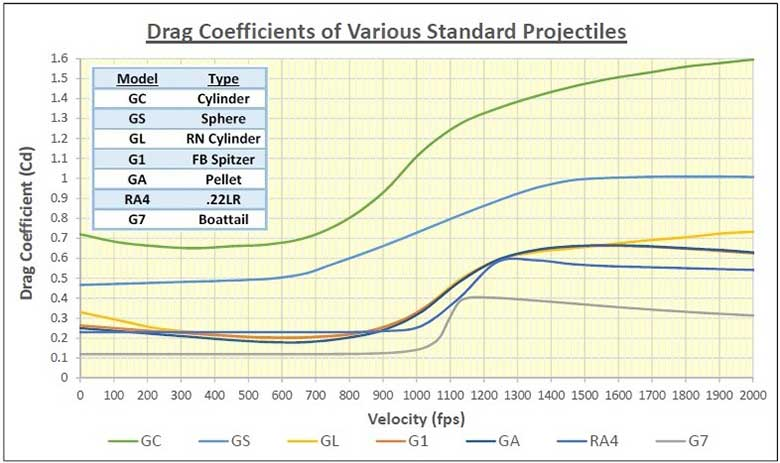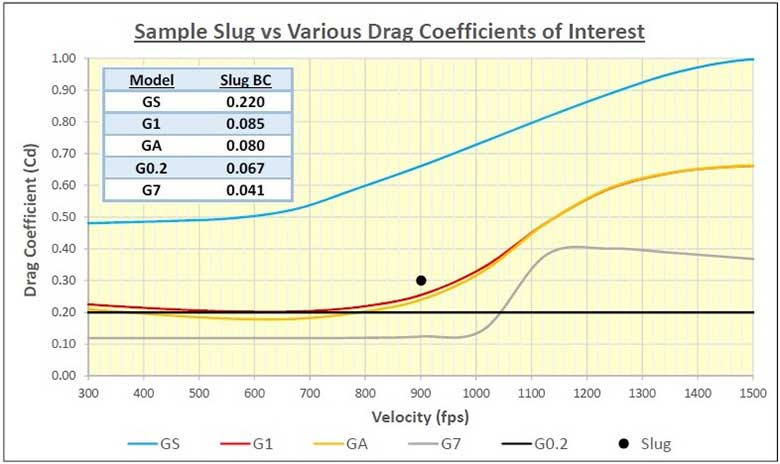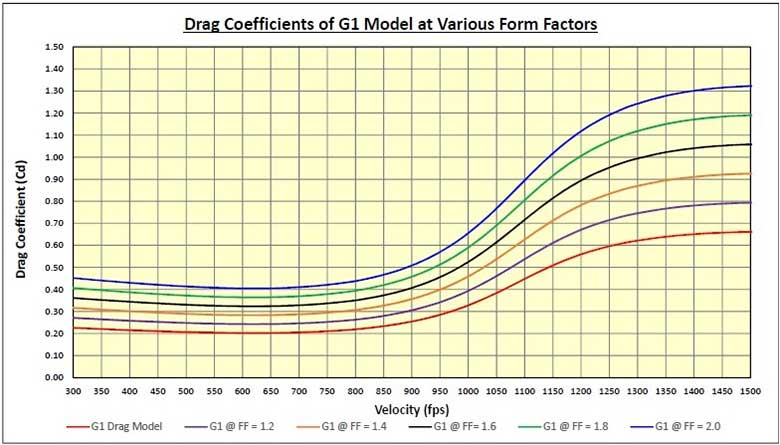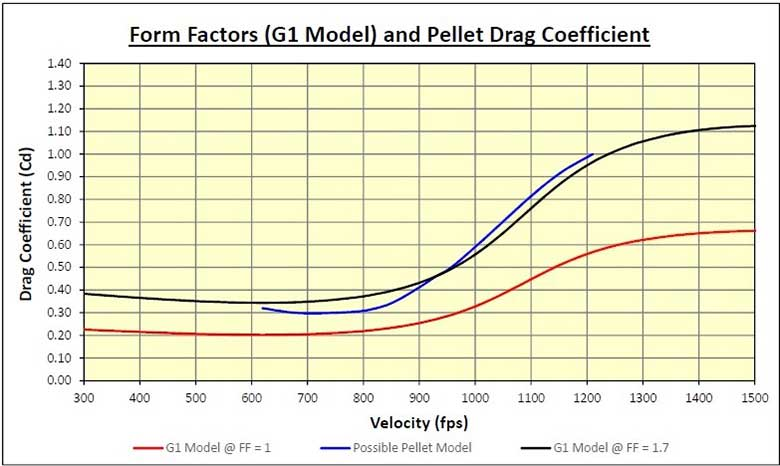# Drag Coefficient and Ballistics Coefficient Explained

Throughout my articles I have many times referred to the terms Drag Coefficient (Cd) and Ballistics Coefficient (BC). In this article I will attempt to explain the difference between them, and how they are related to each other.

The Cd is a measure of the drag on an object. It changes depending on the velocity. While relatively constant at low speeds, when you approach the speed of sound (Mach 1) the drag increases rapidly. In the Transonic range (Mach 0.8-1.2) there is a huge increase in drag for any object, which leads to the term “Sound Barrier” because it takes much more power to drive an object faster than the speed of sound.

The actual Cd, and how it changes with velocity, depends on the shape of the object. A blunt object, like a cylinder, has a lot of drag, while a streamlined object like a boattail bullet has much less drag. However, they both experience a huge relative increase in drag between Subsonic flight (under Mach 0.8) and Supersonic flight (over Mach 1.2).

Here is a chart showing how the Cd changes relative to the velocity for several shapes:Note the large increase in drag above Mach 0.8 (900 fps). The shapes used in the chart above are so called “Standard Drag Models”. The ones of the most interest to us are the GS (sphere or roundball), the G1 (used for most flat based bullets), the GA (used for airgun pellets), and sometimes the G7 (used for long, pointed boattail bullets).

##### The Old “Constant Drag Model”

Airgun pellets were sometimes compared to a “constant drag model”, typically using a Cd of 0.2, regardless of the velocity. This is sometimes still used, and there is at least one online calculator for airgun ballistics that does so. While it isn’t bad below about 900 fps, it diverges drastically from the real world above that.

The earliest version of ChairGun used it, which is why some of the early work I did showing the BC changing radically as the velocity increased had the results they did. I measured BCs on some pellets that dropped by a factor of three (eg. from 0.036 to 0.012) between 800 fps and 1100 fps. This was a representation of what the drag did, but a BC that varies like that is useless.

The constant drag model was at fault, and ChairGun moved from that to using the G1 model, and then to the newer GA model. Each has been an improvement, and currently the GA is the best standard drag model we have for Diabolo pellets. The G1 model is most often used for calculating the BC of slugs, however.

##### BC Depends on Drag Model Chosen

The “Form Factor” (FF) is calculated by dividing the Cd of your projectile by the Cd of the constant drag model, at the velocity the Cd’s were measured at. A FF of less than 1 indicates your projectile has less drag than the standard projectile at that velocity. If the FF is greater than 1, your projectile has more drag at that velocity. You will get a different value for the FF, depending on the standard drag model you choose, and the velocity.

The BC is calculated by dividing the Sectional Density (SD) of your projectile by the FF. Therefore, you will also get a different answer for the BC, depending on the drag model chosen. Consider a slug with an SD of 0.10, and a Cd of 0.30 at 900 fps, represented by the black dot in this chart:Note that each of the standard drag models have a different Cd at 900 fps. This means that the calculated BC will vary, depending on which drag model you used. The small table in the corner of the chart shows the BC of our sample slug, using each drag model, at 900 fps.

If your projectile matches the standard drag model closely, your real world results will correspond closely to theory. If you use a drag model that is not suitable, your trajectory and particularly the wind drift, will not be very close to that calculated.

When you quote the BC of a projectile, ideally you should also quote the drag model and the velocity. For this example, the best match would be to quote the ballistics coefficient as BC(G1) = 0.085 at 900 fps. A pellet of the same caliber that weighed half as much (SD = 0.05) but with the same Cd and velocity, should be described as having a BC(GA) = 0.040 at 900 fps, since BC is proportional to SD.

The Ballistics Coefficient values listed by HAM, as measured using a LabRadar unit, are all calculated using the GA drag model, and the velocity is stated. Kudos to the HAM team for this clarity!

##### The Form Factor and Drag Curves

What does the form factor mean? It compares the drag of your slug to the drag of the standard drag model. For a given Sectional Density, the higher the FF, the lower the ballistics coefficient. Remember, BC = SD/FF. Consider the chart below, which shows what happens to the G1 drag model at various form factors:As an example, for an FF = 1.6, you get the black line above. The Cd is 60% greater than that of the standard G1 model at every velocity. When you use the G1 drag model to calculate your BC at one velocity, and then use a ballistics calculator to get the trajectory and wind drift of your slug at other velocities, you are assuming that the Cd is following one of this family of curves, or something in between.

The problem is, rarely is that the case. Several researchers (including myself) have done enough measuring of actual pellet drag to realize that even the GA model is not very accurate in the Transonic range (above Mach 0.8). While we still need a lot more data, it appears that the drag increase above 900 fps for Diabolo pellets is greater than any of the current drag models allow for. Here is an example:The black line on the chart above is what we would get by using the G1 drag profile and an FF of 1.7, a reasonable compromise. However, some of the measurements we have made indicate that the Cd of many pellets is less below 900 fps, and greater above that, with a much more drastic rise in drag than any of the current drag models. The blue line above might be more representative.

The same thing can be said for many of the shapes we use for slugs. The G1 drag model is for a flat based Spitzer (pointed) bullet, whereas most of the slugs we use for airguns have a Meplat (flat nose) or Hollowpoint that is significantly blunter than the drag standard we are using.

There is almost no data at the present time, but I believe that many of those slugs will follow a curve not unlike that blue line, although perhaps a lower Cd everywhere, because the drag of the blunt nose increases much more rapidly than the G1 model in the Transonic range.

Next month I will continue talking about the evolution of the Ballistics Coefficient for both pellets and slugs. With the advent of the LabRadar doppler chronograph, over the next few years you can expect much better drag models to be developed for airgun use, both for Diabolo pellets, and for the flat-nosed or hollowpoint slugs we typically use.